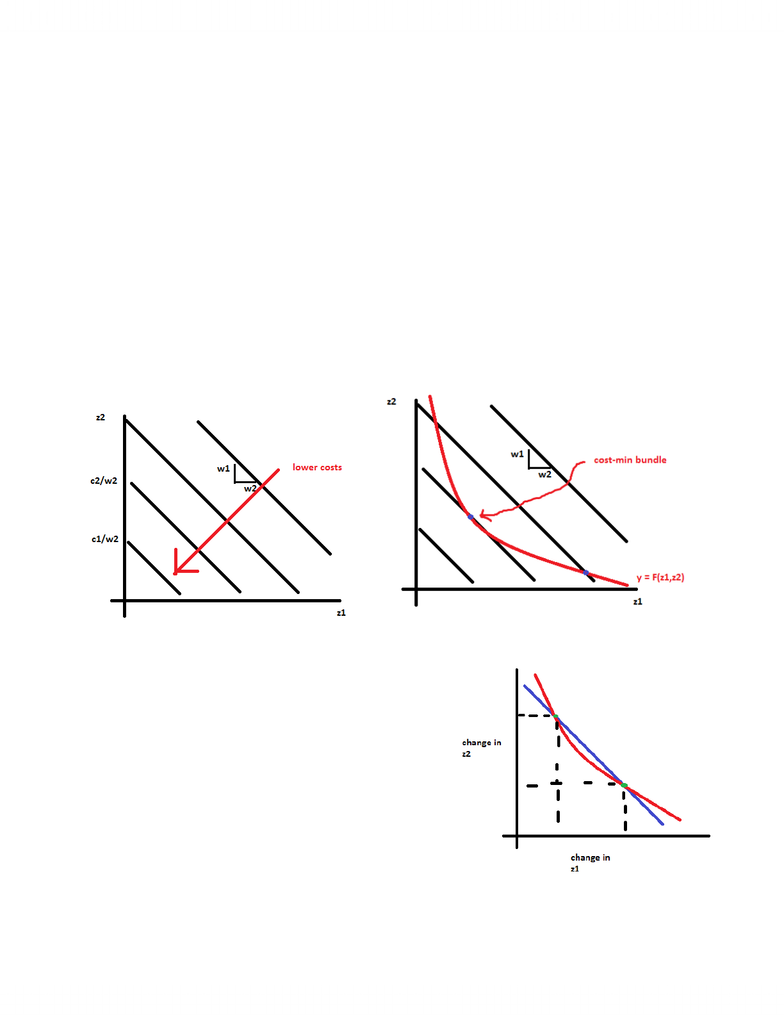# ECON 2X03 Lecture Notes - Lecture 2: Isocost, Zirconium, Bzip2

46 views4 pages
School
McMaster University
Department
Economics
Course
ECON 2X03
ProfessorLecture 2
Long Run Cost Minimization
- Long run: all inputs variable
- Cost minimization: selecting quantities of the inputs to minimize costs given a particular output
- Zi = input i
- Y = output
- Wi = cost per unit of input i
- Min(z1,z2) w1z1 +w2z2
o Subject to F(z1,z2) = y
- Consider firms with diminishing MRTS:
o Assume that from the firm;s perspective, the cost per unit of inputs is fixed, the cost (c)
of some input bundle is C = w1z1 + w2z2
o Isocost: a line along which the input bundles have the same cost (FIXED)
o C = w1z1 + w2z2
o Z2 = c/w2 + w1*z1/w2
o Cost-min where:
slope of isoquant = slope of isocost
MRTS = w1/w2
- MP1 = Δy/ Δz1 Δz1 = Δy1/MP1
- MP2 = Δy/ Δz2 Δz2 = Δy2/MP2
- MRTS = -Δz2/Δz1 = (-Δy2/MP2)/( Δy1/MP1)
- MRTS = (-Δy2/MP2)*(MP1/Δy) = -
(Δy2/Δy1)*(MP1/MP2)
- Since Δy2 = -Δy1 ( since back to same isoquant):
o MRTS = MP1/MP2
With diminishing MRTS:
- Cost-min condition is MP1/MP2 = w1/w2
Unlock document

This preview shows page 1 of the document.
Unlock all 4 pages and 3 million more documents.